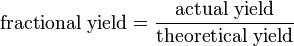# Yield (chemistry) facts for kids

Kids Encyclopedia Facts

In chemistry, yield (also called chemical yield or reaction yield) is the amount of product resulting from a chemical reaction. The absolute yield gives the weight in grams, and the molar yield gives the number of moles.

The fractional yield, relative yield, or percentage yield shows how completely a synthetic procedure worked. It is calculated by dividing the amount of product by the theoretical yield (the unit of measurement for both must be the same):$\mbox{fractional yield} = \frac{\mbox{actual yield}}{\mbox{theoretical yield}}$

To get the percentage yield, one must multiply the fractional yield by 100% (e.g., 0.673 × 100% = 67.3%).

The ideal percentage yield of a chemical reaction is 100%, but it cannot be achieved because measurements are never completely accurate. Yields around 100% are "quantitative", yields above 90% are "excellent", yields above 80% are "very good", yields above 70% are "good", yields below 50% are "fair",however yields below 40% are "poor".Yield (chemistry) Facts for Kids. Kiddle Encyclopedia.